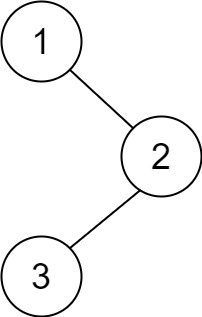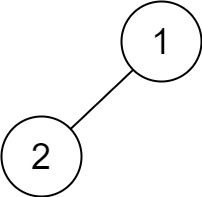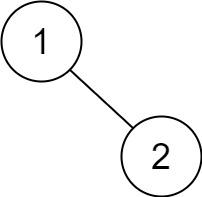# LeetCode Notes: Binary Tree Postorder Traversal

## Question

Given the `root` of a binary tree, return the postorder traversal of its nodes' values.

Example 1:Input: root = [1,null,2,3]

Output: [3,2,1]

Example 2:

Input: root = []

Output: []

Example 3:

Input: root = 

Output: 

Example 4:Input: root = [1,2]

Output: [2,1]

Example 5:Input: root = [1,null,2]

Output: [2,1]

Constraints:

• The number of the nodes in the tree is in the range `[0, 100]`.
• `-100 <= Node.val <= 100`

Follow up: Recursive solution is trivial, could you do it iteratively?

## Solution

Analysis:

Recursively visit the left subtree, right subtree, and root node of the binary tree, and store them in an array.

Code:

``````/**
* Definition for a binary tree node.
* function TreeNode(val, left, right) {
* this.val = (val===undefined? 0: val)
* this.left = (left===undefined? null: left)
* this.right = (right===undefined? null: right)
*}
*/
/**
* @param {TreeNode} root
* @return {number[]}
*/
var postorderTraversal = function (root) {
// Special case
if (root == null) return [];

// Recursively, to access the left subtree, right subtree, root node, and store the node value in the array
function postOrder(node, result = []) {
if (node != null) {
postOrder(node.left, result);
postOrder(node.right, result);
result.push(node.val);
}
}
// Create a new array for storing the results and start recursively traversing the entire binary tree
const result = [];
postOrder(root, result);
return result;
};
``````